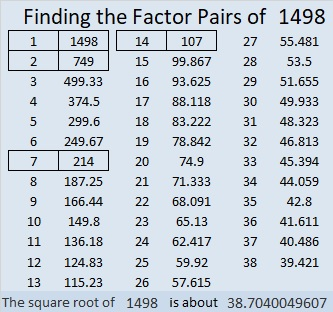# 1498 Another Mystery

Contents

### Today’s Puzzle:

The number 48 appears four times in a 12 × 12 multiplication table, and all four 48’s appear in this puzzle! Where will you put its factors, 6, 8, 4, 12? Use logic to figure out where all the numbers from 1 to 12 need to go to make this puzzle turn into a multiplication table.### Factors of 1498:

• 1498 is a composite number.
• Prime factorization: 1498 = 2 × 7 × 107.
• 1498 has no exponents greater than 1 in its prime factorization, so √1498 cannot be simplified.
• The exponents in the prime factorization are 1, 1, and 1. Adding one to each exponent and multiplying we get (1 + 1)(1 + 1)(1 + 1) = 2 × 2 × 2 = 8. Therefore 1498 has exactly 8 factors.
• The factors of 1498 are outlined with their factor pair partners in the graphic below.### Pythagorean Triples with 1498:

1498 is 2(107)(7) so we can calculate a cool Pythagorean triple from
2(107)(7), 107² – 7², 107² + 7² to get 1498-11400-11498.

Also from 2(749)(1), 749² – 1², 749² + 1², we get 1498-561000-561002.

This site uses Akismet to reduce spam. Learn how your comment data is processed.